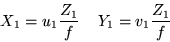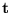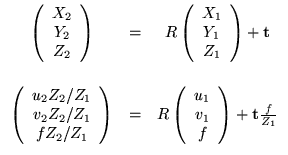Next: Batch Techniques Up: 3D Structure from 2D Previous: Small Baselines

# Nonlinear Approaches

Many nonlinear frameworks can be related to the classic Relative Orientation problem proposed by Horn  . The technique is a two-frame one with a perspective projection camera model. Structure and motion (but not camera internal geometry) are recovered by minimizing a nonlinear cost function.

The technique begins with a setup similar to the one in Figure 4 without any of the epipolar details. In addition, the focal length f is assumed to be given. Assume that the point P is actually represented as unknown 3D coordinates P1 and P2 in two different coordinate systems (one for each COP). These 3D coordinates are directly related to their 2D projections (u1,v1) and (u2,v2) by perspective projection as in Equation 7.(7)

The projection of P in one image plane can be defined as a translation and rotation of the 3D point in the coordinate system of the other. Thus, the 3D point P1 in COP1 can be computed from the 3D point P2 in COP2 as in Equation 8. Here, rotation R and translationdefine the relative 3D motion between the two cameras.(8)

Each corresponded pair of points increases the system by three more equations with two more unknowns (the Z1 and Z2). The system can undergo an arbitrary scaling so a normality constraint is applied to translation to force a unique solution. In addition, there are orthonormality constraints on the rotation matrix (which only has 3 true degrees of freedom). Thus, the 5 unknowns for the relative 3D motion can be solved using 5 point correspondences. However, a least-squares version with more points is preferred for accuracy. The solution minimizes error using iterative nonlinear optimization. In the process, the values of Z1 and Z2 are solved for each corresponded point and 3D structure is recovered.Next: Batch Techniques Up: 3D Structure from 2D Previous: Small Baselines

1999-05-17